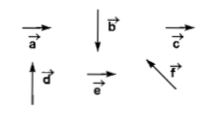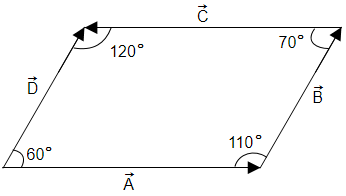Component of $3\stackrel{^}{i}+4\stackrel{^}{j}$ perpendicular to $\stackrel{^}{i}+\stackrel{^}{j}$ and in the same plane as that of $3\stackrel{^}{i}+4\stackrel{^}{j}$ is:

1. $\frac{1}{2}\left(\stackrel{^}{j}-\stackrel{^}{i}\right)$

2. $\frac{3}{2}\left(\stackrel{^}{j}-\stackrel{^}{i}\right)$

3. $\frac{5}{2}\left(\stackrel{^}{j}-\stackrel{^}{i}\right)$

4. $\frac{7}{2}\left(\stackrel{^}{j}-\stackrel{^}{i}\right)$

Subtopic:  Scalar Product |
To view explanation, please enrol in the course below.
NEET 2021 - Achiever Batch - Aryan Raj Singh

Difficulty Level:

Given that  makes an angle . Which of the following options is correct?

1.

2.

3.

4.

Subtopic:  Resultant of Vectors |
To view explanation, please enrol in the course below.
NEET 2021 - Achiever Batch - Aryan Raj Singh

Difficulty Level:

If the angle between the vector  is  $\theta$, the value of the product $\left(\stackrel{\to }{B}×\stackrel{\to }{A}\right).\stackrel{\to }{A}$ is equal to:

1.

2.

3.

4. zero

Subtopic:  Vector Product |
To view explanation, please enrol in the course below.
NEET 2021 - Achiever Batch - Aryan Raj Singh

Difficulty Level:

Two forces A and B have a resultant ${R}_{1}$. If B is doubled, the new resultant ${R}_{2}$ is perpendicular to A. Then

1. ${R}_{1}=A$

2. ${R}_{1}=B$

3. ${R}_{2}=A$

4. ${R}_{2}=B$

Subtopic:  Resultant of Vectors |
To view explanation, please enrol in the course below.
NEET 2021 - Achiever Batch - Aryan Raj Singh

Difficulty Level:

Choose any one of the following four responses:

1. If both assertion and reason are true and the reason is the correct explanation of the assertion.

2. If both assertion and reason are true but the reason is not the correct explanation of the assertion.

3. If the assertion is true but the reason is false.

4. If the assertion and reason both are false.

Assertion: The graph between P and Q is a straight line when P/Q is constant.

Reason: The straight-line graph means that P proportional to Q or P is equal to constant multiplied by Q.

Subtopic:  Co-ordinate geometry |
To view explanation, please enrol in the course below.
NEET 2021 - Achiever Batch - Aryan Raj Singh

Difficulty Level:

Two forces are such that the sum of their magnitudes is 18 N and their resultant is perpendicular to the smaller force and magnitude of resultant is 12 N. Then the magnitudes of the forces are

1. 12 N, 6 N

2. 13 N, 5N

3. 10 N, 8 N

4. 16 N, 2 N

Subtopic:  Resultant of Vectors |
To view explanation, please enrol in the course below.
NEET 2021 - Achiever Batch - Aryan Raj Singh

Difficulty Level:

If the magnitude of the sum of two vectors is equal to the magnitude of the difference of the two vectors, the angle between these vectors is:

Subtopic:  Resultant of Vectors |

Difficulty Level:

If the magnitude of sum of two vectors is equal to the magnitude of difference of the two vectors, the angle between these vectors is

1. 90°

2. 45°

3. 180°

4. 0°

Subtopic:  Resultant of Vectors |
To view explanation, please enrol in the course below.
NEET 2021 - Achiever Batch - Aryan Raj Singh

Difficulty Level:

Six vectors  have the directions indicated in the figure. Which of the following statements may be true?(a) $\stackrel{\to }{b}+\stackrel{\to }{c}=\stackrel{\to }{-f}$                        (b) $\stackrel{\to }{d}+\stackrel{\to }{c}=\stackrel{\to }{f}$

(c) $\stackrel{\to }{d}+\stackrel{\to }{e}=\stackrel{\to }{f}$                        (d) $\stackrel{\to }{b}+\stackrel{\to }{e}=\stackrel{\to }{f}$

Subtopic:  Resultant of Vectors |
To view explanation, please enrol in the course below.
NEET 2021 - Achiever Batch - Aryan Raj Singh

Difficulty Level:

In the given figure(1)  Angle between $\stackrel{\to }{\mathrm{A}}$ and $\stackrel{\to }{\mathrm{B}}$ is $110°$

(2)  Angle between $\stackrel{\to }{\mathrm{C}}$ and $\stackrel{\to }{\mathrm{D}}$ is $60°$

(3)  Angle between $\stackrel{\to }{\mathrm{B}}$ and $\stackrel{\to }{\mathrm{C}}$ is $110°$

(4)  Angle between $\stackrel{\to }{\mathrm{B}}$ and $\stackrel{\to }{\mathrm{C}}$ is $70°$

Subtopic:  Resultant of Vectors |
To view explanation, please enrol in the course below.
NEET 2021 - Achiever Batch - Aryan Raj Singh

Difficulty Level: